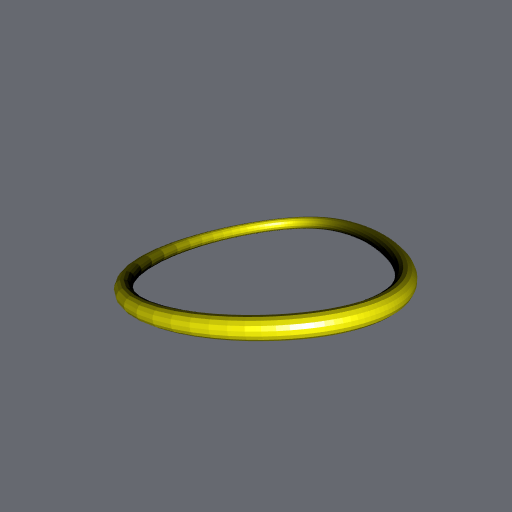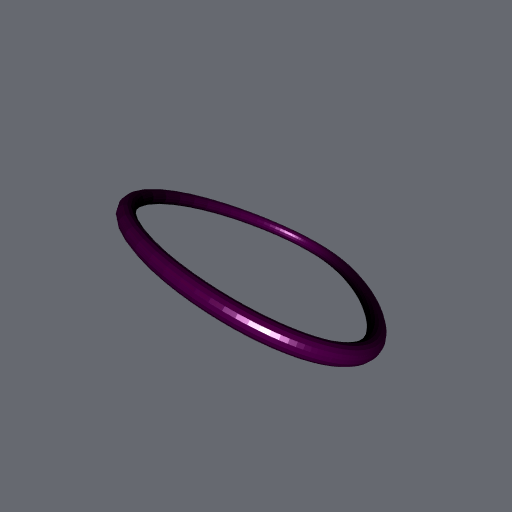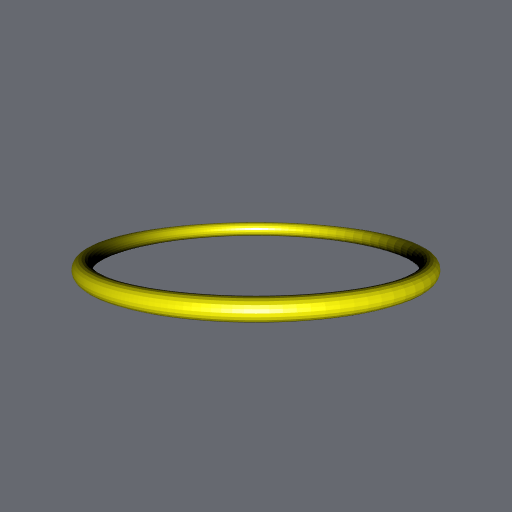Want to share your content on R-bloggers? click here if you have a blog, or here if you don't.

Remember my first post on the Hopf torus? I constructed it circle by circle. Below are some animations of this construction. I save the image each time a circle is added. The rgl package automatically centers the plot, and this gives a nice effect.

First animation, three lobes, using a modified stereographic projection:Here is the code producing this animation:

```# Hopf fiber
HopfFiber <- function(q, t){
1/sqrt(2*(1+q[1L])) * c(q[3L]*cos(t) + q[2L]*sin(t),
q[2L]*cos(t) - q[3L]*sin(t),
sin(t)*(1+q[1L]),
cos(t)*(1+q[1L]))
}
# Modified stereographic projection
mstereog <- function(x){
acos(x[4L])/sqrt(1-x[4L]^2) * x[1L:3L]
}

# plot
library(rgl)
open3d(windowRect = c(50, 50, 562, 562))
bg3d("#666970")
view3d(0, 0, zoom = 0.9)
t_ <- seq(0, 2*pi, len = 200L) # 200 subdivisions per circle
u_ <- seq(0, 2*pi, len = 300L) # 300 circles
nlobes <- 3L # number of lobes of the Hopf torus
colors <- colorRampPalette( # colors
interpolate = "spline", bias = 0.15
)(150L)
colors <- c(colors, rev(colors))
for(i in 1:length(u_)){
u <- u_[i]
x <-  cos(pi/2 - (pi/2-0.44)*cos(nlobes*u))
z <-  sin(pi/2 - (pi/2-0.44)*cos(nlobes*u)) * cos(u+0.44*sin(2*nlobes))
y <- -sin(pi/2 - (pi/2-0.44)*cos(nlobes*u)) * sin(u+0.44*sin(2*nlobes))
circle4d <- vapply(t_, function(t){
HopfFiber(c(x, y, z), t)
}, numeric(4L))
circle3d <- t(apply(circle4d, 2L, mstereog))
cylinder3d(circle3d, radius = 0.1, sides = 15),
color = colors[i]
)
rgl.snapshot(sprintf("pic%03d.png", i)) # save
}

# duplicate last pic to make a pause at the end of the animation
for(i in 301L:350L){
file.copy("pic300.png", sprintf("pic%03d.png", i))
}
# make animation
pngFiles <- list.files(pattern = "^pic?.*png\$")
library(gifski)
gifski(
pngFiles,
gif_file = "HopfTorusCircleByCircle_3lobes.gif",
width    = 512, height   = 512,
delay    = 1/9 # 9 pics per second
)
# delete png files
file.remove(pngFiles)```

Four lobes, modified stereographic projection, with the ‘rocket’ color palette (in grDevices package):Two lobes, classical stereographic projection: